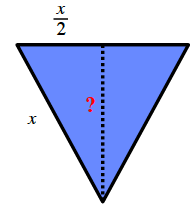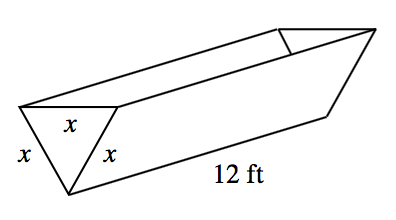### Home > PC3 > Chapter 3 > Lesson 3.1.2 > Problem3-33

3-33.

A trough is in the shape of an equilateral triangular prism, as shown in the diagram at right. The length of the trough is $12$ feet.

1. Write an equation for the height of the equilateral triangle in terms of $x$.

Draw and label the triangle with information you know. Use the Pythagorean Theorem to solve for the height.

$\frac{\sqrt{3}}{2}x$2. Write an equation for the volume $V$ of the trough in terms of $x$.

$V(x)=$ (area of the triangle)(length of the trough)

3. If the trough can hold $200\text{ ft}^3$ of water, how deep is the trough?

Solve $200=V(x)$.